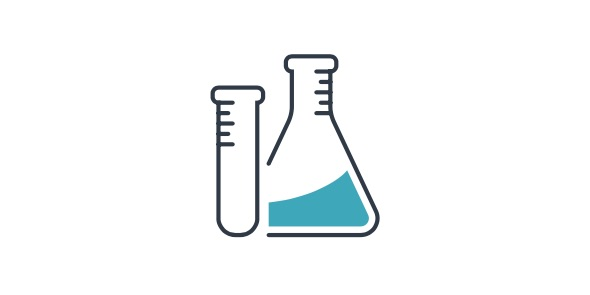# Science Quiz

17 Questions | Total Attempts: 48SettingsThis is a quiz for cbse 8th std board.

Related Topics
• 1.
1) What is atomicity?
• A.

It is the number of atoms present in a molecule of an element.

• B.

It is the number of molecules in an atom.

• C.

It is the number of protons in an atom

• D.

It is the number of atoms in an atom

• 2.
Molecules like helium, argon and neon contains only one atom. They are
• A.

Mono atomic molecules

• B.

Poly atomic molecules

• C.

Di-atomic molecules

• D.

Tri-atomic molecules

• 3.
If the molecule consists of atoms of same type it is called molecule of an element
• A.

True

• B.

False

• 4.
It the molecule contains atoms of two or more elements it is called a molecule of an compoud.
• A.

True

• B.

False

• 5.
If a molecule consists of only one atom it is called poly atomic molecule.
• A.

False

• B.

True

• 6.
Molecules like hydrogen,oxygen and nitrogen contains only two atoms. They are
• A.

Mono atomic molecules

• B.

Poly atomic molecules

• C.

Di-atomic molecules

• D.

Tri-atomic molecules

• 7.
Molecules like Ozone contains three atoms. They are
• A.

Mono atomic molecules

• B.

Poly atomic molecules

• C.

Di-atomic molecules

• D.

Tri-atomic molecules

• 8.
Molecules like phosporous contains four atoms. They are
• A.

Mono atomic molecules

• B.

Poly atomic molecules

• C.

Di-atomic molecules

• D.

Tri-atomic molecules

• 9.
The inner most shell of an atom is called valence shell.
• A.

True

• B.

False

• 10.
The electrons in the inner most shell is called valence shell
• A.

True

• B.

False

• 11.
The number of protons and electorns are not same in an atom
• A.

True

• B.

False

• 12.
The number of protons is equal to the number of electrons in an atom
• A.

True

• B.

False

• 13.
The atomic number is the number of protons in an atoms.
• A.

True

• B.

False

• 14.
The atomic number is not the number of protons or electrons in an atom
• A.

True

• B.

False

• 15.
THE MASS NUMBER IS THE NUMBER OF PROTONS IN AN ATOM.
• A.

True

• B.

False

• 16.
THE MASS NUMBER IS THE NUMBER OF electrons IN AN ATOM (F)
• A.

True

• B.

False

• 17.
THE MASS NUMBER IS THE NUMBER OF PROTONS and neutrons IN AN ATOM
• A.

True

• B.

False